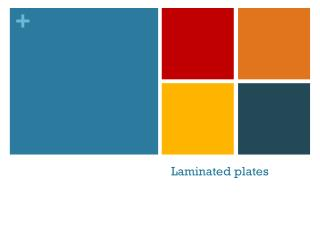DownloadDownload PresentationLaminated plates

Laminated plates

Télécharger la présentationLaminated plates

- - - - - - - - - - - - - - - - - - - - - - - - - - - E N D - - - - - - - - - - - - - - - - - - - - - - - - - - -
Presentation Transcript

1. Laminated plates

2. definitions • Unidirectional: orientation direction is identical for all laminates • Angle-ply: • Fiber orientation alternates between +theta to –theta from the stress normal • …/q/-q/q/-q/… • Cross ply: • Fibers in alternating plies are at right angles to each other • …/0/90/0/90/… • Symmetric: fiber orientation in the stack is symmetric about the center line of the laminate

3. Symmetric laminate examples • 7 ply stack: • This stack is symmetrical about the centerline of the 4th ply • The stack order is a ‘mirror’ reflection about the centerline • We will need the average height of the ply above the centerline.

4. Symmetric laminate examples • 8 ply stack: • This stack is symmetrical about the interface between ply 4 and ply 5 • The stack order is a ‘mirror’ reflection about the centerline • We will need the average height of the ply above the centerline.

5. Antisymmetric • Ply orientation is antisymmetric about the centerline of the laminate • For each ply with fiber orientation angle, q, above the midplane, this is a ply of fiber orientation angle, –q, with identical material and thickness and equal distance below the midplane • q(z)=-q(-z) • q/-q/q/-q is antisymmetric • q/-q/-q/q is symmetric

6. Unsymmetric laminate • Unsymmetric: q1/q2/q3; random placement of angles • Quasi-isotropic laminate: equal angles between adjacent lamina, increment is p/n • +60/0/-60 • +45/0/-45/-90 • 0/+60/-60 • 0/+45/-45/90

7. Quasi-isotropic laminates • Quasi-isotropic laminate: equal angles between adjacent lamina, increment is p/n • +60/0/-60 • +45/0/-45/-90 • 0/+60/-60 • 0/+45/-45/90 • A common quasi-isotropic symmetrical stacking sequence is: [0/±45/90]s

8. Code examples • [0/45/90]s: 1st angle is for the outermost ply, last angle is for the innermost ply; s subscript means symmetrical • The bar over 90 indicates that the plan of symmetry passes midway through the thickness of the 90 degree laminate • ±45 implies adjacent +45 and -45 degrees laminates • 04: four adjacent zero degree plies • (±45)2: two grouped, adjacent ±45 degree plies

9. In-class examples • Sketch the following: • [0/45/90]s • [0/-45/90]s • [0/±45/90]s

10. Young B35 classroom (30 student seats and 1 teacher station)                 Thursday 4/3 3:30pm-4:45pm                 Thursday 4/10 3:30pm-4:45pm Peggy M. Akridge Manager, Student Computing Services UK Analytics and Technologies University of Kentucky Lamination theory

11. Step-by-step procedure • Calculate stiffness matrices for the laminate • Calculate the midplane strains and curatures for the laminate due to specific applied forces • Calculate in-plane stresses, exx, eyy, gxy, for each lamina • Calculate in-plane strains, sxx, syy, txy, for each lamina

12. Assumptions • Laminate is thin, wide: w>>t • Perfect interlaminar bonds • Strain distribution in the thickness direction is linear • All laminas are macroscopically homogeneous and behave linearly elastically

13. Laminate strains • Since strain distribution in z direction is linear, • Geometric midplane = xy axes, z axis defines thickness direction • Total laminate thickness = h • Thickness of various lamina are ti • Total lamina = N

14. Laminate strains • Since strain distribution in z direction is linear, • e0ii = midplane normal strains • g0xy = midplane shear strain • Kiibending curvatures of laminate • Kxy = twisting curvature of laminate